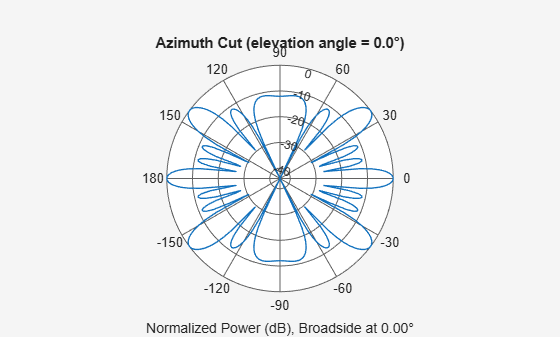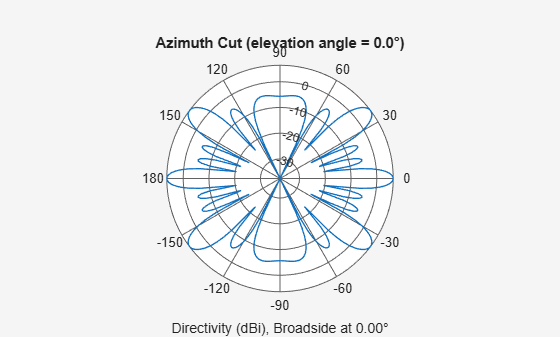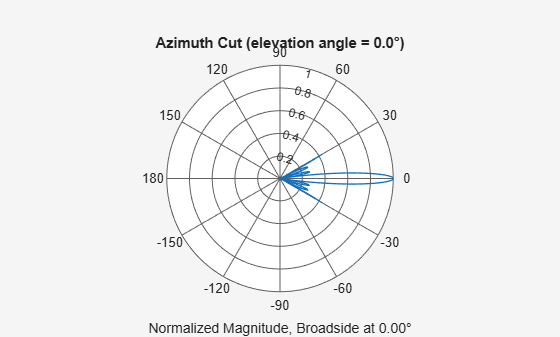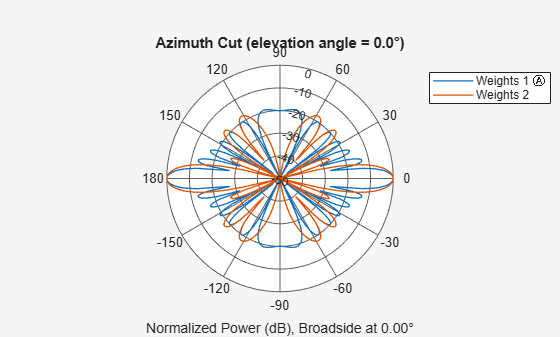# plotResponse

System object: phased.ReplicatedSubarray
Package: phased

Plot response pattern of array

## Syntax

```plotResponse(H,FREQ,V) plotResponse(H,FREQ,V,Name,Value) hPlot = plotResponse(___) ```

## Description

`plotResponse(H,FREQ,V)` plots the array response pattern along the azimuth cut, where the elevation angle is 0. The operating frequency is specified in `FREQ`. The propagation speed is specified in `V`.

`plotResponse(H,FREQ,V,Name,Value)` plots the array response with additional options specified by one or more `Name,Value` pair arguments.

`hPlot = plotResponse(___)` returns handles of the lines or surface in the figure window, using any of the input arguments in the previous syntaxes.

## Input Arguments

 `H` Array object. `FREQ` Operating frequency, in hertz. Typical values are within the range specified by a property of `H.Subarray.Element`. That property is named `FrequencyRange` or `FrequencyVector`, depending on the type of element in the array. The element has zero response at frequencies outside that range. If `FREQ` is a nonscalar row vector, the plot shows multiple frequency responses on the same axes. `V` Propagation speed in meters per second.

### Name-Value Arguments

Specify optional pairs of arguments as `Name1=Value1,...,NameN=ValueN`, where `Name` is the argument name and `Value` is the corresponding value. Name-value arguments must appear after other arguments, but the order of the pairs does not matter.

Before R2021a, use commas to separate each name and value, and enclose `Name` in quotes.

`CutAngle`

Cut angle specified as a scalar. This argument is applicable only when `RespCut` is `'Az'` or `'El'`. If `RespCut` is `'Az'`, `CutAngle` must be between –90 and 90. If `RespCut` is `'El'`, `CutAngle` must be between –180 and 180.

Default: `0`

`Format`

Format of the plot, using one of `'Line'`, `'Polar'`, or `'UV'`. If you set `Format` to `'UV'`, `FREQ` must be a scalar.

Default: `'Line'`

`NormalizeResponse`

Set this value to `true` to normalize the response pattern. Set this value to `false` to plot the response pattern without normalizing it. This parameter is not applicable when you set the `Unit` parameter value to `'dbi'`.

Default: `true`

`OverlayFreq`

Set this value to `true` to overlay pattern cuts in a 2-D line plot. Set this value to `false` to plot pattern cuts against frequency in a 3-D waterfall plot. If this value is `false`, then `FREQ` must be a vector with at least two entries.

This parameter applies only when `Format` is not `'Polar'` and `RespCut` is not `'3D'`.

Default: `true`

`Polarization`

Specify the polarization options for plotting the array response pattern. The allowable values are ```|'None' | 'Combined' | 'H' | 'V' |``` where:

• `'None'` specifies plotting a nonpolarized response pattern

• `'Combined'` specifies plotting a combined polarization response pattern

• `'H'` specifies plotting the horizontal polarization response pattern

• `'V'` specifies plotting the vertical polarization response pattern

For arrays that do not support polarization, the only allowed value is `'None'`. This parameter is not applicable when you set the `Unit` parameter value to `'dbi'`.

Default: `'None'`

`RespCut`

Cut of the response. Valid values depend on `Format`, as follows:

• If `Format` is `'Line'` or `'Polar'`, the valid values of `RespCut` are `'Az'`, `'El'`, and `'3D'`. The default is `'Az'`.

• If `Format` is `'UV'`, the valid values of `RespCut` are `'U'` and `'3D'`. The default is `'U'`.

If you set `RespCut` to `'3D'`, `FREQ` must be a scalar.

`SteerAng`

Subarray steering angle. `SteerAng` can be either a 2-element column vector or a scalar.

If `SteerAng` is a 2-element column vector, it has the form [azimuth; elevation]. The azimuth angle must be between –180 and 180 degrees, inclusive. The elevation angle must be between –90 and 90 degrees, inclusive.

If `SteerAng` is a scalar, it specifies the azimuth angle. In this case, the elevation angle is assumed to be 0.

This option is applicable only if the `SubarraySteering` property of `H` is `'Phase'` or `'Time'`.

Default: `[0;0]`

`Unit`

The unit of the plot. Valid values are `'db'`, `'mag'`, `'pow'`, or `'dbi'`. This parameter determines the type of plot that is produced.

Unit valuePlot type
`db`power pattern in dB scale
`mag`field pattern
`pow`power pattern
`dbi`directivity

Default: `'db'`

`Weights`

Weight values applied to the array, specified as a length-N column vector or N-by-M matrix. The dimension N is the number of subarrays in the array. The interpretation of M depends upon whether the input argument `FREQ` is a scalar or row vector.

Weights DimensionFREQ DimensionPurpose
N-by-1 column vectorScalar or 1-by-M row vectorApply one set of weights for the same single frequency or all M frequencies.
N-by-M matrixScalarApply all of the M different columns in `Weights` for the same single frequency.
1-by-M row vectorApply each of the M different columns in `Weights` for the corresponding frequency in `FREQ`.

`AzimuthAngles`

Azimuth angles for plotting subarray response, specified as a row vector. The `AzimuthAngles` parameter sets the display range and resolution of azimuth angles for visualizing the radiation pattern. This parameter is allowed only when the `RespCut` parameter is set to `'Az'` or `'3D'` and the `Format` parameter is set to `'Line'` or `'Polar'`. The values of azimuth angles should lie between –180° and 180° and must be in nondecreasing order. When you set the `RespCut` parameter to `'3D'`, you can set the `AzimuthAngles` and `ElevationAngles` parameters simultaneously.

Default: `[-180:180]`

`ElevationAngles`

Elevation angles for plotting subarray response, specified as a row vector. The `ElevationAngles` parameter sets the display range and resolution of elevation angles for visualizing the radiation pattern. This parameter is allowed only when the `RespCut` parameter is set to `'El'` or `'3D'` and the `Format` parameter is set to `'Line'` or `'Polar'`. The values of elevation angles should lie between –90° and 90° and must be in nondecreasing order. When you set the `RespCut` parameter to `'3D'`, you can set the `ElevationAngles` and `AzimuthAngles` parameters simultaneously.

Default: `[-90:90]`

`UGrid`

U coordinate values for plotting subarray response, specified as a row vector. The `UGrid` parameter sets the display range and resolution of the U coordinates for visualizing the radiation pattern in U/V space. This parameter is allowed only when the `Format` parameter is set to `'UV'` and the `RespCut` parameter is set to `'U'` or `'3D'`. The values of `UGrid` should be between –1 and 1 and should be specified in nondecreasing order. You can set the `UGrid` and `VGrid` parameters simultaneously.

Default: `[-1:0.01:1]`

`VGrid`

V coordinate values for plotting subarray response, specified as a row vector. The `VGrid` parameter sets the display range and resolution of the V coordinates for visualizing the radiation pattern in U/V space. This parameter is allowed only when the `Format` parameter is set to `'UV'` and the `RespCut` parameter is set to `'3D'`. The values of `VGrid` should be between –1 and 1 and should be specified in nondecreasing order. You can set the `VGrid` and `UGrid` parameters simultaneously.

Default: `[-1:0.01:1]`

## Examples

expand all

Plot the azimuth response of a 4-element ULA composed of two 2-element ULAs.

Create a 2-element ULA, and arrange two copies to form a 4-element ULA.

```h = phased.ULA('NumElements',2,'ElementSpacing',0.5); ha = phased.ReplicatedSubarray('Subarray',h,... 'Layout','Rectangular','GridSize',[1 2],... 'GridSpacing','Auto');```

Plot the azimuth response of the array. Assume the operating frequency is 1 GHz and the wave propagation speed is 3e8 m/s.

`plotResponse(ha,1e9,3e8,'RespCut','Az','Format','Polar');`Plot the azimuth directivity of the array.

`plotResponse(ha,1e9,3e8,'RespCut','Az','Format','Polar','Unit','dbi');`Create a 2-element ULA, and arrange two copies to form a 4-element ULA. Using the `AzimuthAngles` parameter, plot the response within a restricted range of azimuth angles from -30 to 30 degrees in 0.1 degree increments.

```h = phased.ULA('NumElements',2,'ElementSpacing',0.5); ha = phased.ReplicatedSubarray('Subarray',h,... 'Layout','Rectangular','GridSize',[1 2],... 'GridSpacing','Auto'); plotResponse(ha,1e9,3e8,'RespCut','Az','Format','Polar',... 'AzimuthAngles',[-30:0.1:30],'Unit','mag');```Construct an array of replicated subarrays. Start with a 2-element uniform line array (ULA), and duplicate it 5 times to create a 10-element ULA. Apply both uniform weights and tapered weights. Then, use `plotResponse` to show that the tapered set of weights reduces the adjacent sidelobes while broadening the main lobe.

```h = phased.ULA('NumElements',2,'ElementSpacing',0.2); ha = phased.ReplicatedSubarray('Subarray',h,... 'Layout','Rectangular','GridSize',[1 5],... 'GridSpacing',0.4); c = physconst('LightSpeed'); fc = 1e9; wts1 = [0.2,0.2,0.2,0.2,0.2]'; wts2 = [0.1,0.23333,.33333,0.23333,0.1]'; plotResponse(ha,fc,c,'RespCut','Az','Format','Polar',... 'Weights',[wts1,wts2]);```